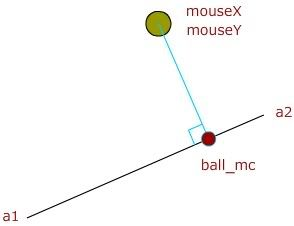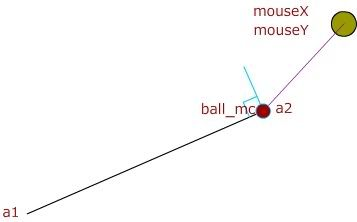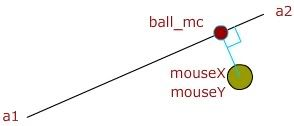A Flash Developer Resource Site

# Thread: as3 mathmatic help!

1. ## as3 mathmatic help!I'm trying to make the red ball(ball_mc) stay in that line between a1 & a2
and I want is position to be relative to the mouseX,MouseY in a perpendicular way(90 degrees).Reply With Quote

2. here you go.Reply With Quote

3. Can you give me some code example.Reply With Quote

4. PHP Code:
``` public static function getClosestPointOnLineSegment( a:Point, b:Point, point:Point ) : Point{        //vector pointing from a to b    //all valid points on the given line segment satisfy a + diff * t for 0 <= t <= 1    var diff:Point = b.subtract( a );    //vector pointing from a to point    var pa:Point = point.subtract( a );    //project the point onto diff- divide by the squared magnitude of diff to solve for t    var t:Number = ( diff.x * pa.x + diff.y * pa.y ) / ( diff.x * diff.x + diff.y * diff.y );    //working with a line segment, ensure t doesn't exceed legal limits    t = Math.max( Math.min( 1, t ), 0 );        return new Point( a.x + diff.x * t, a.y + diff.y * t );    }  ```
a and b are the 2 endpoints of the line segment, point is the center of your circle. you'll have to import flash.geom.Point.

the link realMakc posted should be sufficient to understand the algorithm.Reply With Quote

5. thanks, sorry, I'm new with the package thing.
I saved your code in ".as" file as linear.as
___________________
1012: The static attribute may be used only on definitions inside a class.

var th_class:linear= new linear()

any help.Reply With Quote

6. if you're just writing the function in Flash, lose public and static (just keep function)Reply With Quote

7. newblack of course you do realize that by giving code you do not help... /unsubReply With Quote

8. I learn better in actionscript.Reply With Quote

9.Originally Posted by realMakc
newblack of course you do realize that by giving code you do not help... /unsub
that's pretty insulting considering i tried my best to comment the code such that it explains why and how it works...Reply With Quote

10. It took me some time but I understand it now.
THANKS NEWBLACK!Reply With Quote

11. you want the ball to change as the mouse's x position changes.

ball_mc.x=mouse.x;

but you want it in-between a1 and a2 which are have pixel locations, that you can find.

a1.x=pixel location#
a1.y=pixel location#

a2.x=pixel location#
a2.y= pixel location#

Your code may have somethig like this.

if(mouse.x<=a2.x){...}
else if(mouse.x>=a1.x){...}
else{}

Also, you may want to look at slope intercep form of a line:

y=mx+bReply With Quote

12. You can work out the bugs of this. I did it quickly:
var p=element ("dot");
var p1=element ("point1");
var p2=element ("point2");

//y=mx+b (equation of a line)

//slope = rise/run

function move(evt:Event):void
{
var slope:Number = ((p2.y)-(p1.y))/((p2.x)-(p1.x))

p.x=mouseX

p.y = slope * p.x +400
}

the "+400" part should varyReply With Quote

####Posting Permissions

• You may not post new threads
• You may not post replies
• You may not post attachments
• You may not edit your posts
•

 » Home » Movies » Tutorials » Submissions » Board » Links » Reviews » Feedback » Gallery » Fonts » The Lounge » Sound Loops » Sound FX » About FK » Sitemap

Click Here to Expand Forum to Full Width